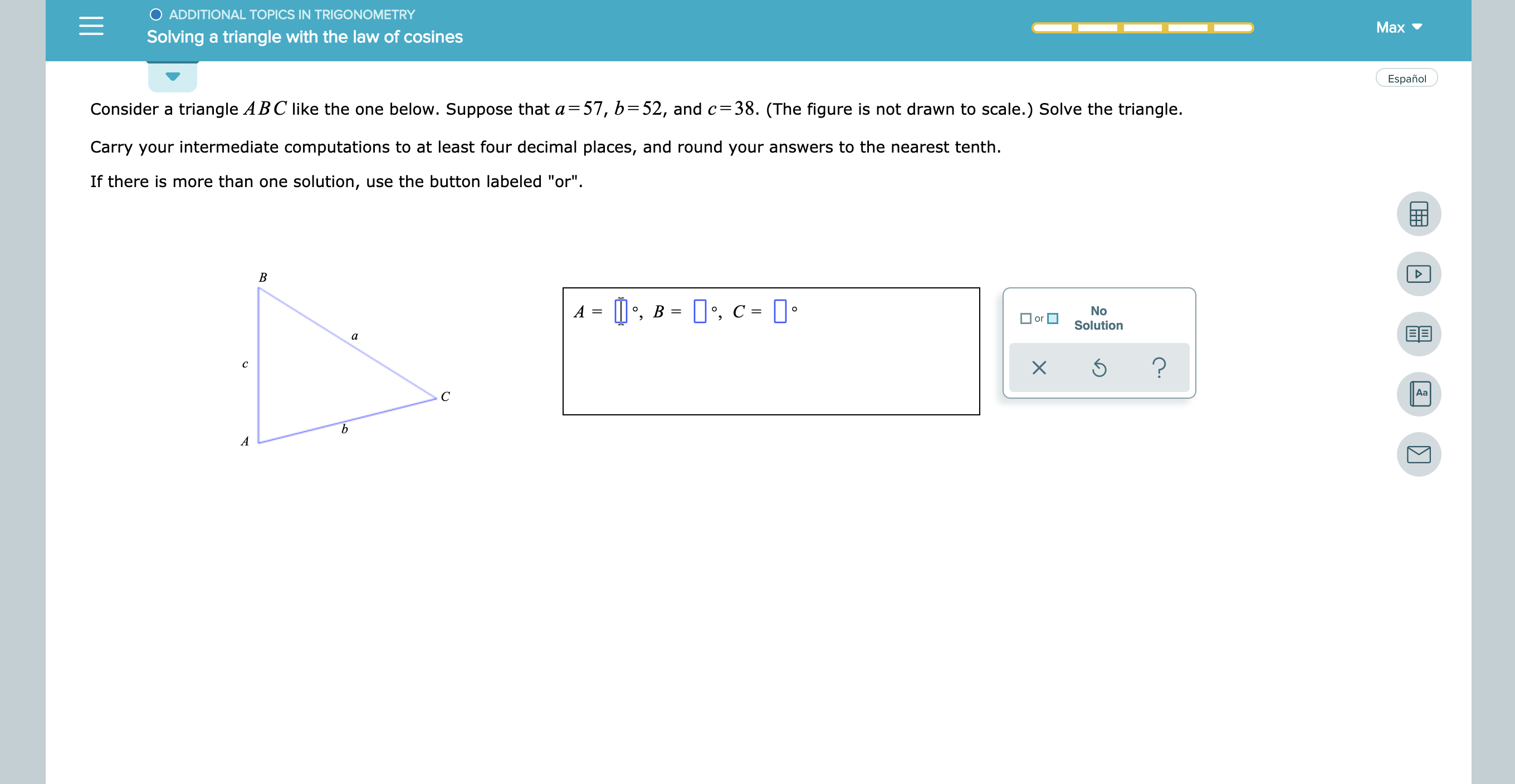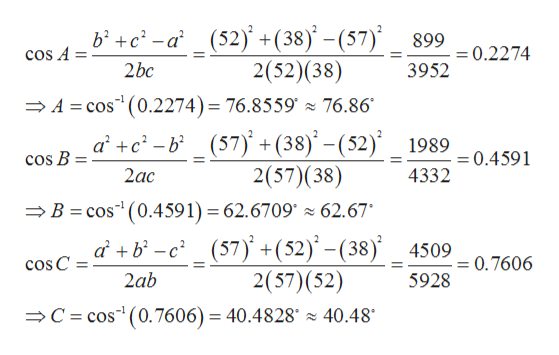# O ADDITIONAL TOPICS IN TRIGONOMETRYMaxSolving a triangle with the law of cosinesEspañoltriangle ABC like the one below. Suppose that a- 57, b=52, and c=38. (The figure is not drawn to scale.) Solve the triangle.Consider aCarry your intermediate computations to at least four decimal places, and round your answers to the nearest tenthIf there is more than one solution, use the button labeled "or"B,B , C-NoOorSolutionBEа?cAaС

Question
63 views

see attachmenthelp_outlineImage TranscriptioncloseO ADDITIONAL TOPICS IN TRIGONOMETRY Max Solving a triangle with the law of cosines Español triangle ABC like the one below. Suppose that a- 57, b=52, and c=38. (The figure is not drawn to scale.) Solve the triangle. Consider a Carry your intermediate computations to at least four decimal places, and round your answers to the nearest tenth If there is more than one solution, use the button labeled "or" B ,B , C- No O or Solution BE а ? c Aa С fullscreen
check_circle

Step 1

Given,

Step 2

By using law of cos...help_outlineImage Transcriptionclosebc -a _(52) +(38) - (57) _ 2(52)(38) 899 = 0.2274 cos A 2bc 3952 A cos(0.2274) = 76.8559 76.86 a +c-b(57) +(38) -(52) 2(57)(38) 1989 0.4591 COs B 4332 2ac B cos (0.4591) 62.6709 z 62.67* (57) +(52)-(38) 2(57) (52) b- 4509 = 0.7606 cos C 2ab 5928 C cos(0.7606) = 40.4828 40.48 fullscreen

### Want to see the full answer?

See Solution

#### Want to see this answer and more?

Solutions are written by subject experts who are available 24/7. Questions are typically answered within 1 hour.*

See Solution
*Response times may vary by subject and question.
Tagged in

### Other